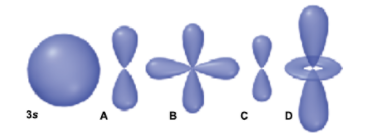# Problem: Use the relative size of the 3s orbital represented below to answer the following question.In an atom, how many other orbitals will have both a value of n and a shape identical to Orbital C? (Use Orbital C as a reference and do not include it in your count.)a. 1b. 2c. 3d. 4

###### FREE Expert Solution
87% (459 ratings)
###### FREE Expert Solution
87% (459 ratings)###### Problem Details

Use the relative size of the 3s orbital represented below to answer the following question.

In an atom, how many other orbitals will have both a value of n and a shape identical to Orbital C? (Use Orbital C as a reference and do not include it in your count.)

a. 1

b. 2

c. 3

d. 4What scientific concept do you need to know in order to solve this problem?

Our tutors have indicated that to solve this problem you will need to apply the Quantum Numbers: Angular Momentum Quantum Number concept. You can view video lessons to learn Quantum Numbers: Angular Momentum Quantum Number. Or if you need more Quantum Numbers: Angular Momentum Quantum Number practice, you can also practice Quantum Numbers: Angular Momentum Quantum Number practice problems.

What is the difficulty of this problem?

Our tutors rated the difficulty ofUse the relative size of the 3s orbital represented below to...as low difficulty.

How long does this problem take to solve?

Our expert Chemistry tutor, Sabrina took 3 minutes and 32 seconds to solve this problem. You can follow their steps in the video explanation above.

What professor is this problem relevant for?

Based on our data, we think this problem is relevant for Professor Amateis' class at VT.